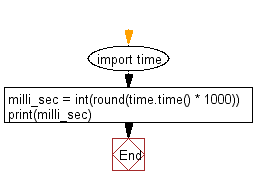﻿ Python: Get current time in milliseconds - w3resource

# Python: Get current time in milliseconds

## Python datetime: Exercise-12 with Solution

Write a Python program to get the current time in milliseconds in Python.

Sample Solution:

Python Code:

``````import time
milli_sec = int(round(time.time() * 1000))
print(milli_sec)
```
```

Sample Output:

```1494055960573
```

Flowchart:Python Code Editor: